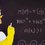# Have you ever realized that you have forgot the boundary conditions?

Once in a while, you will forget about the boundary conditions. Today, I am going to be talking about the Time dependent Schrodinger Equation for a particle in a box as an example to show that boundary conditions really matter when solving certain equations. For those who aren't familiar with the Time dependent Schrodinger Equation, it follows: $i\hbar \frac { \partial \Psi }{ \partial t } =-\frac { \hbar ^{ 2 } }{ 2m } \nabla ^{ 2 }\Psi +V(x)\Psi$. The boundary conditions of the box are $0\le x\le L$. We could solve the Schrodinger Equation using separation of variables so we get $\Psi (x,t)=Asin(kx)$ but if we forgot about the boundary conditions, we would get $\Psi (x,t)=Ae^{ i(kr-\omega t) }$. We would also get different energy eigenvalues. For $\Psi (x,t)=Asin(kx)$, we would get ${ E }_{ n }=\frac { \hbar ^{ 2 }n^{ 2 }\pi ^{ 2 } }{ 2mL^{ 2 } }$ whereas, for $\Psi (x,t)=Ae^{ i(kr-\omega t) }$, you would get that there are no certain energy eigenvalues. In conclusion, always remember to include boundary conditions in your courses such as differential equations and other courses. I am 12 and many people in my school really don't care about the conditions that are given anyways so that is why I am posting this.Note by Sunny Sahu
7 years ago

This discussion board is a place to discuss our Daily Challenges and the math and science related to those challenges. Explanations are more than just a solution — they should explain the steps and thinking strategies that you used to obtain the solution. Comments should further the discussion of math and science.

When posting on Brilliant:

• Use the emojis to react to an explanation, whether you're congratulating a job well done , or just really confused .
• Ask specific questions about the challenge or the steps in somebody's explanation. Well-posed questions can add a lot to the discussion, but posting "I don't understand!" doesn't help anyone.
• Try to contribute something new to the discussion, whether it is an extension, generalization or other idea related to the challenge.

MarkdownAppears as
*italics* or _italics_ italics
**bold** or __bold__ bold
- bulleted- list
• bulleted
• list
1. numbered2. list
1. numbered
2. list
Note: you must add a full line of space before and after lists for them to show up correctly
paragraph 1paragraph 2

paragraph 1

paragraph 2

[example link](https://brilliant.org)example link
> This is a quote
This is a quote
    # I indented these lines
# 4 spaces, and now they show
# up as a code block.

print "hello world"
# I indented these lines
# 4 spaces, and now they show
# up as a code block.

print "hello world"
MathAppears as
Remember to wrap math in $$ ... $$ or $ ... $ to ensure proper formatting.
2 \times 3 $2 \times 3$
2^{34} $2^{34}$
a_{i-1} $a_{i-1}$
\frac{2}{3} $\frac{2}{3}$
\sqrt{2} $\sqrt{2}$
\sum_{i=1}^3 $\sum_{i=1}^3$
\sin \theta $\sin \theta$
\boxed{123} $\boxed{123}$

Sort by:

I use this for challenging questions but I am listed under 42 years old for some reason.

- 7 years ago